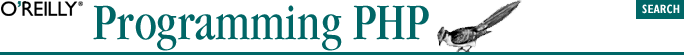home | O'Reilly's CD bookshelfs | FreeBSD | Linux | Cisco | Cisco Exam## 5.5. Extracting Multiple Values

`list(\$variable, ...) = \$array;`

The array's values are copied into the listed variables, in the array's internal order. By default that's the order in which they were inserted, but the sort functions described later let you change that. Here's an example:

```\$person = array('name' => 'Fred', 'age' => 35, 'wife' => 'Betty');
list(\$n, \$a, \$w) = \$person;            // \$n is 'Fred', \$a is 35, \$w is 'Betty'```

If you have more values in the array than in the list( ), the extra values are ignored:

```\$person = array('name' => 'Fred', 'age' => 35, 'wife' => 'Betty');
list(\$n, \$a) = \$person;                // \$n is 'Fred', \$a is 35```

If you have more values in the list( ) than in the array, the extra values are set to NULL:

```\$values = array('hello', 'world');
list(\$a, \$b, \$c) = \$values;            // \$a is 'hello', \$b is 'world', \$c is NULL```
```\$values = range('a', 'e');
list(\$m,,\$n,,\$o) = \$values;            // \$m is 'a', \$n is 'c', \$o is 'e'```